GRAVITY
I seek to describe gravity without assuming anything but the motion of the aether.

• I assume no action-at-distance (matter "attracting" matter instantly over space).
• I assume no "gravitational fields" that are part of space, but not space, and not matter.
• I assume no "bending" of "space-time."
• I assume no extra dimensions beyond that which we can immediately verify (three).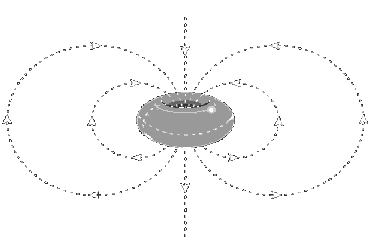What is Gravity?What is the origin of a gravitational system?
A gravity system beings with an extreme local disturbance in the aether-medium. Current theories suggest
that, with regard to our solar system, this might have been due to a supernova explosion.
This is the nebular
hypothesis.
Regardless of the exact cause we just need to know that "gases" (the aether can be modeled
after an ideal gas)
under tremendous turbulent motion tend to gather angular momentum--that is, begin to
spin or rotate. Rotational motion achieves the greatest equilibrium under turbulent conditionsThe Kinematic Evolution of a vortex in a three-dimensional fluid
 Any vortex in a three-dimensional medium takes the shape of a torus.
At the center of any torus vortex there is an area of low pressure called a "sink." This is represented by the
depression in the torus.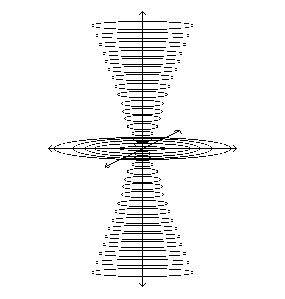The dynamic pressure, or high velocity, of the vortex means that the aether that passes through
the sink perpendicular to the vortex is "back-reflected." Furthermore, the aether (in the case of the
depiction below) "above" the vortex is reflected into the sink. This causes a series of loops as
shown below.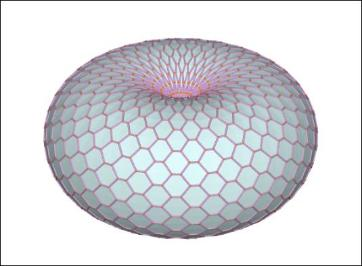A vortex in a three-dimensional medium is formally called:

A Spiral Torus Sink Vortex with Accompanying Sink-Source Flow

The figures below show of the sink-source flow the "conical-source" and "conical-sink" flows (static figure) as well as
the "loop-flow" (animation); as well as the vortex itself.
These flow patterns are unified units of motion as described by
Bernoulli's Principle.
The next page describes the vector structure of the torus vortex.A: Solenoid Conical through-flow
B: Loop-flow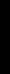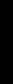Contents

•                What is not assumed.
•                What is the origin of gravity?
•                The kinematics of a vortex in three dimensions: an explanation in figures
•                        The vortex
•                        The sink-source flow

Gravity Part II
•                The kinematics of a vortex in three dimensions: an in explanation in words

Gravity Part III
•                The application of the spiral torus vortex with sink-source flow to the phenomenon of gravity
•                        A gravity system's magnetic field explained
•                        A gravity system's radial "force" explained

Gravity Part IV
•                The further application of the spiral torus vortex with sink-source flow to the phenomenon of gravity
•                        A gravity system's tangential force (why it orbits a body and why bodies orbit it)
•                        Why the star or planet at the center of a gravity system expands (expanding earth)

Gravity Part V
•                Visual empirical evidence of the spiral torus vortex (photographs of galaxies)Source: Aethro-Kinematics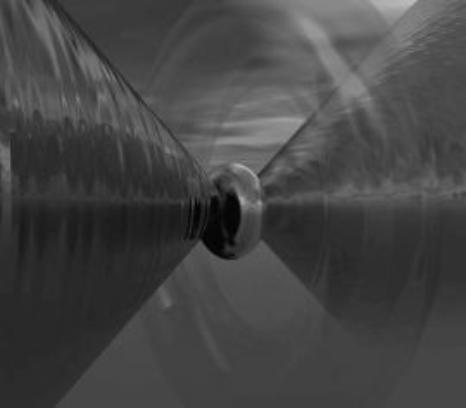"Step 1": The Spiral Torus Vortex
The sink, the area of low pressure, or low density, or rarefied space, passes through the vortex perpendicular to
its plane of motion. This area of low pressure is a density disturbance of the aether. To equilibrate the
disturbance non-vortex aether will flow into it, from either above or below, flow through it, and flow out of it.
This "sink-to-source flow" takes two forms: a) solenoid conical through-flow and b) loop-flow
 "Step 2": The Sink at the Center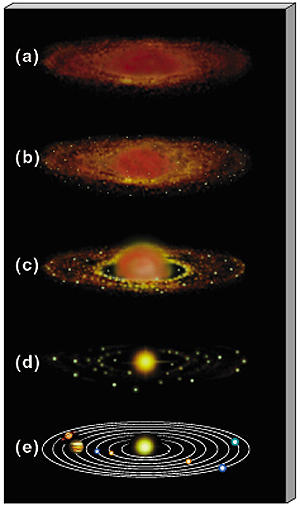"Step 3": Fluid Flow In and Out of the Sink
The aether spirals into and out of the center of the sink of the vortex. It spirals because, as it enters the area of
the vortex, the latter imparts on the formers its rotational motion.
 "Step 4": A Unified Unit of Motion